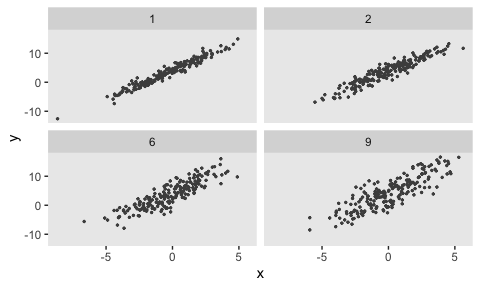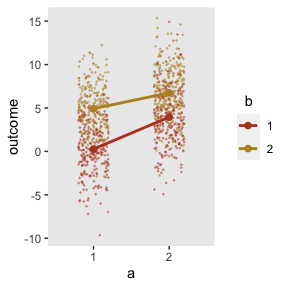# Dynamic Data Definition

Often, we’d like to explore data generation and modeling under different scenarios. For example, we might want to understand the operating characteristics of a model given different variance or other parametric assumptions. There is functionality built into simstudy to facilitate this type of dynamic exploration. First, the functions updateDef and updateDefAdd essentially allow us to edit lines of existing data definition tables. Second, there is a built-in mechanism - called double-dot reference - to access external variables that do not exist in a defined data set or data definition.

## Updating existing definition tables

The updateDef function updates a row in a definition table created by functions defData or defRead. Analogously, updateDefAdd function updates a row in a definition table created by functions defDataAdd or defReadAdd.

The original data set definition includes three variables x, y, and z, all normally distributed:

defs <- defData(varname = "x", formula = 0, variance = 3, dist = "normal")
defs <- defData(defs, varname = "y", formula = "2 + 3*x", variance = 1, dist = "normal")
defs <- defData(defs, varname = "z", formula = "4 + 3*x - 2*y", variance = 1, dist = "normal")

defs
##    varname       formula variance   dist     link
## 1:       x             0        3 normal identity
## 2:       y       2 + 3*x        1 normal identity
## 3:       z 4 + 3*x - 2*y        1 normal identity

In the first case, we are changing the relationship of y with x as well as the variance:

defs <- updateDef(dtDefs = defs, changevar = "y", newformula = "x + 5", newvariance = 2)
defs
##    varname       formula variance   dist     link
## 1:       x             0        3 normal identity
## 2:       y         x + 5        2 normal identity
## 3:       z 4 + 3*x - 2*y        1 normal identity

In this second case, we are changing the distribution of z to Poisson and updating the link function to log:

defs <- updateDef(dtDefs = defs, changevar = "z", newdist = "poisson", newlink = "log")
defs
##    varname       formula variance    dist     link
## 1:       x             0        3  normal identity
## 2:       y         x + 5        2  normal identity
## 3:       z 4 + 3*x - 2*y        1 poisson      log

And in the last case, we remove a variable from a data set definition. Note in the case of a definition created by defData that it is not possible to remove a variable that is a predictor of a subsequent variable, such as x or y in this case.

defs <- updateDef(dtDefs = defs, changevar = "z", remove = TRUE)
defs
##    varname formula variance   dist     link
## 1:       x       0        3 normal identity
## 2:       y   x + 5        2 normal identity

## Double-dot external variable reference

For a truly dynamic data definition process, simstudy (as of version 0.2.0) allows users to reference variables that exist outside of data generation. These can be thought of as a type of hyperparameter of the data generation process. The reference is made directly in the formula itself, using a double-dot (“..”) notation before the variable name. Here is a simple example:

def <- defData(varname = "x", formula = 0,
variance = 5, dist = "normal")
def <- defData(def, varname = "y", formula = "..B0 + ..B1 * x",
variance = "..sigma2", dist = "normal")

def
##    varname         formula variance   dist     link
## 1:       x               0        5 normal identity
## 2:       y ..B0 + ..B1 * x ..sigma2 normal identity
B0 <- 4;
B1 <- 2;
sigma2 <- 9

set.seed(716251)

dd <- genData(100, def)

fit <- summary(lm(y ~ x, data = dd))

coef(fit)
##             Estimate Std. Error t value Pr(>|t|)
## (Intercept)     4.00      0.284    14.1 2.56e-25
## x               2.01      0.130    15.4 5.90e-28
fit$sigma ##  2.83 It is easy to create a new data set on the fly with a difference variance assumption without having to go to the trouble of updating the data definitions. sigma2 <- 16 dd <- genData(100, def) fit <- summary(lm(y ~ x, data = dd)) coef(fit) ## Estimate Std. Error t value Pr(>|t|) ## (Intercept) 4.35 0.427 10.19 4.57e-17 ## x 2.12 0.218 9.75 4.32e-16 fit$sigma
##  4.21

The double-dot notation can be flexibly applied using lapply (or the parallel version mclapply) to create a range of data sets under different assumptions:

sigma2s <- c(1, 2, 6, 9)

gen_data <- function(sigma2, d) {
dd <- genData(200, d)
dd\$sigma2 <- sigma2
dd
}

dd_4 <- lapply(sigma2s, function(s) gen_data(s, def))
dd_4 <- rbindlist(dd_4)

ggplot(data = dd_4, aes(x = x, y = y)) +
geom_point(size = .5, color = "grey30") +
facet_wrap(sigma2 ~ .) +
theme(panel.grid = element_blank())## Using non-scalar double-dot variable reference

The double-dot notation is also array-friendly. For example if we want to create a mixture distribution from a vector of values (which we can also do using a categorical distribution), we can define the mixture formula in terms of the vector. In this case we are generating permuted block sizes of 2 and 4:

defblk <- defData(varname = "blksize",
formula = "..sizes | .5 + ..sizes | .5", dist = "mixture")

defblk
##    varname                           formula variance    dist     link
## 1: blksize ..sizes | .5 + ..sizes | .5        0 mixture identity
sizes <- c(2, 4)
genData(1000, defblk)
##         id blksize
##    1:    1       4
##    2:    2       4
##    3:    3       4
##    4:    4       2
##    5:    5       4
##   ---
##  996:  996       2
##  997:  997       2
##  998:  998       4
##  999:  999       4
## 1000: 1000       4

In this second example, there is a vector variable tau of positive real numbers that sum to 1, and we want to calculate the weighted average of three numbers using tau as the weights. We could use the following code to estimate a weighted average theta:

tau <- rgamma(3, 5, 2)
tau <- tau / sum(tau)
tau
##  0.319 0.550 0.132
d <- defData(varname = "a", formula = 3, variance = 4)
d <- defData(d, varname = "b", formula = 8, variance = 2)
d <- defData(d, varname = "c", formula = 11, variance = 6)
d <- defData(d, varname = "theta", formula = "..tau*a + ..tau*b + ..tau*c",
dist = "nonrandom")

set.seed(1)
genData(4, d)
##    id    a    b    c theta
## 1:  1 1.75 8.47 12.4  6.84
## 2:  2 3.37 6.84 10.3  6.18
## 3:  3 1.33 8.69 14.7  7.13
## 4:  4 6.19 9.04 12.0  8.52

We can simplify the calculation of theta by using matrix multiplication:

d <- updateDef(d, changevar = "theta", newformula = "t(..tau) %*% c(a, b, c)")

set.seed(1)
genData(4, d)
##    id    a    b    c theta
## 1:  1 1.75 8.47 12.4  6.84
## 2:  2 3.37 6.84 10.3  6.18
## 3:  3 1.33 8.69 14.7  7.13
## 4:  4 6.19 9.04 12.0  8.52

These arrays can also have multiple dimensions, as in a $$2 \times 2$$ matrix. If we want to specify the mean outcomes for a factorial study design with two interventions $$a$$ and $$b$$, we can use a simple matrix and draw the means directly from the matrix, which in this example is stored in the variable effect:

effect <- matrix(c(0, 4, 5, 7), nrow = 2)
effect
##      [,1] [,2]
## [1,]    0    5
## [2,]    4    7

Using double dot notation, it is possible to reference the matrix cell values directly:

d1 <- defData(varname = "a", formula = ".5;.5", variance = "1;2", dist = "categorical")
d1 <- defData(d1, varname = "b", formula = ".5;.5", variance = "1;2", dist = "categorical")
d1 <- defData(d1, varname = "outcome", formula = "..effect[a, b]", dist="nonrandom")
dx <- genData(8, d1)
dx
##    id a b outcome
## 1:  1 2 2       7
## 2:  2 2 2       7
## 3:  3 2 1       4
## 4:  4 2 1       4
## 5:  5 1 1       0
## 6:  6 2 2       7
## 7:  7 2 1       4
## 8:  8 1 2       5

It is possible to generate normally distributed data based on these means:

d1 <- updateDef(d1, "outcome", newvariance = 9, newdist = "normal")
dx <- genData(1000, d1)

The plot shows the individual values as well as the mean values by intervention arm:

## Warning: Using size aesthetic for lines was deprecated in ggplot2 3.4.0.
## ℹ Please use linewidth instead.